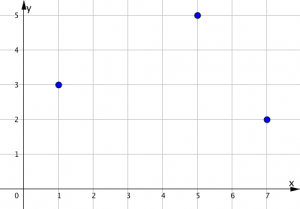# Definitions

A set is a collection of objects. The objects in a set are called elements.

Sets are usually referred to with upper case letters. The elements are usually referred to with lower case letters.

Here are two sets:

P={1, 5, 7}

Q={2, 3, 5}

An ordered pair is when we take two elements and write them in a specific order. For example, (1,3) is an ordered pair.

A relation is a set of ordered pairs. The set R below is a relation, because each element in the set is an ordered pair.

R={(1,3); (5,5); (7,2)}

Because both parts of the ordered pair are numerical, we can draw a diagram of this relation on the xy plane.Notice that the first element in each ordered pair came from the set P. This set is the domain for the relation R.

The second element in each ordered pair comes from the set Q. This set is the range for the relation R.

Some relations, like this one, are really quite random. In other relations, a number pattern can be spotted between the first and second value in the ordered pair. Number patterns quite often lead to shape patterns when the relation is drawn on the xy plane. Indeed, when we draw a relation, this helps us to ‘see’ the number pattern.

Just like we used a number line to learn how to add and subtract, we use the xy plane to learn all the many fascinating and sometimes beautiful properties of number patterns!

# Linear Relations Exploration (i)

(This video may help with using the slider tool)

Reset the applet between tasks. Use the arrow tool to hit the reset icon at the top right.

Geogebra Classic online

# Something Different

There are different kinds of coordinates: the xy plane above is called the cartesian coordinate system.  GPS (global positioning system) uses latitude and longitude coordinates – type in your address (or Ryder Hesjedal Way for Royal Bay Secondary School) to the address box here. Another kind of coordinate system is called polar coordinates. In all three of these systems (cartesian, GPS, polar), there are two values representing different directions or quantities.

Check out these graphs that use the mathematics of polar coordinates. (Coordinate points on circles rather than squares). The example given is. Try changing out the 2 and the 3 for other values. This is just for fun. After learning about sines and cosines, you will have the math to understand whatandare and how these relations betweenandwork.

Done, take me back to the Relations and Functions Menu